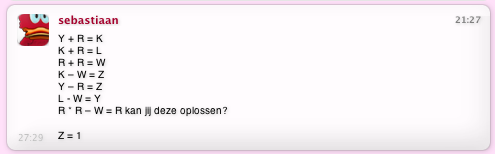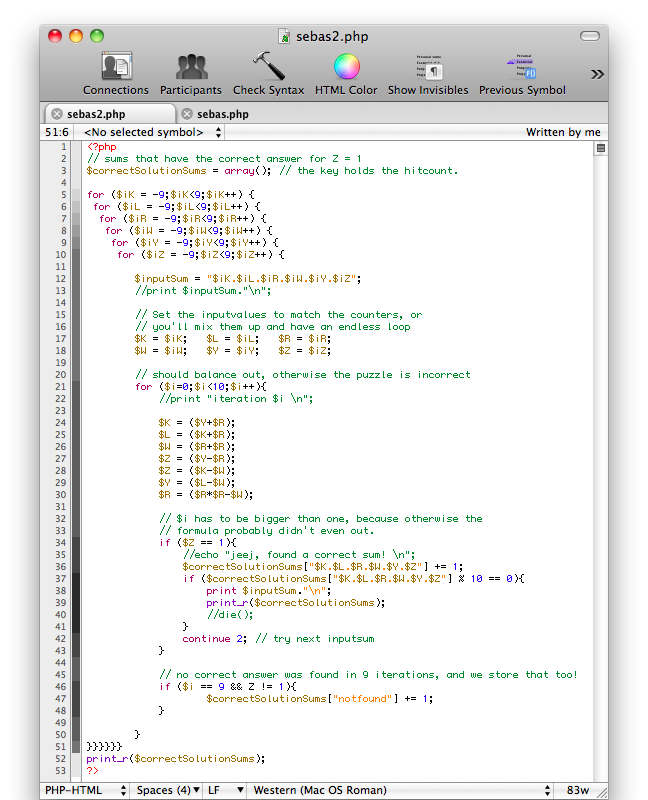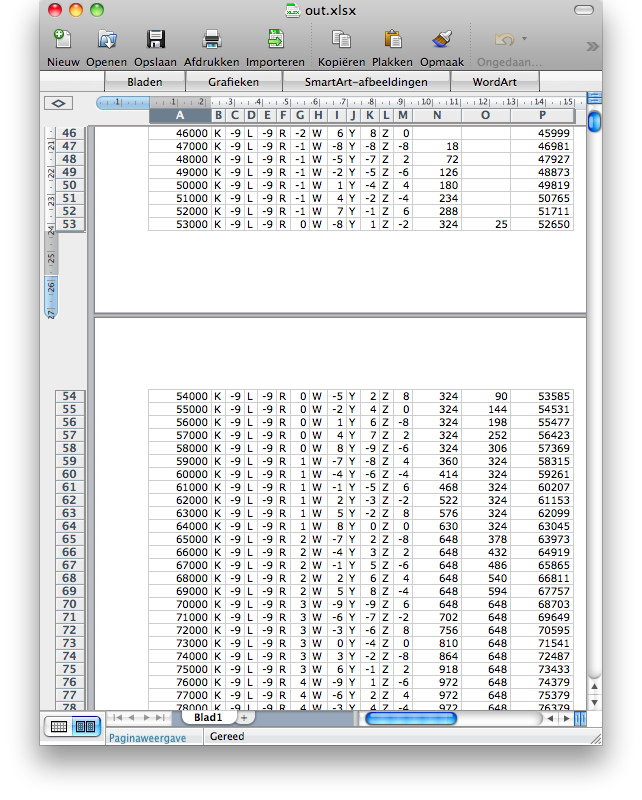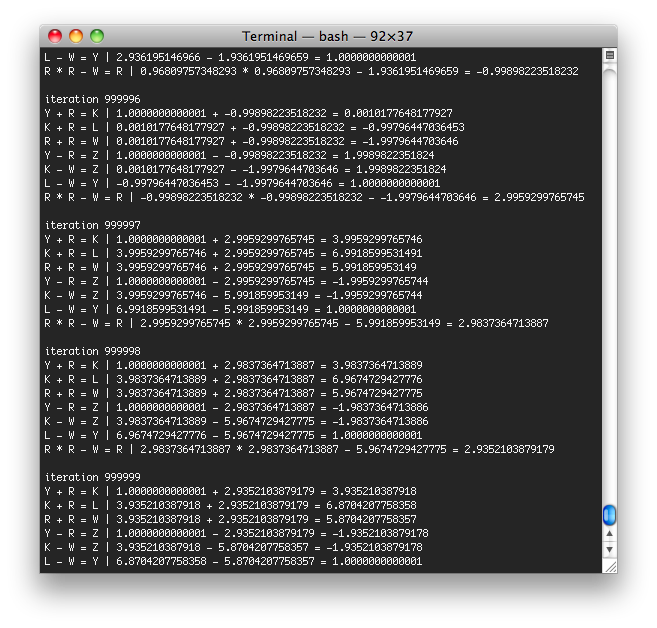# Midnight brainteaser

A friend of mine just sent me a brain teaser. It looked so intriguing, all those numbers ordered in some kind of fashion. So i decided to take on the challenge. This is what Sebastiaan sent:## Taking on the challenge

My first attempt was performed in Textedit, and it was a cumbersome process of shuffling and reshuffling numbers. My guess was that R was a negative number, and with some negative trickery the puzzle was solvable.

After a few minutes of failed attempts I recognized my brain failed at this hour. So lets do some stupid programming, in order to figure out the correct values for the puzzle.

Firing up a text editor and writing out the formula, i hunched at the formula’s tightness. If the formula is not written correctly, the puzzle is incorrect and thus unsolvable. If not, the numbers will probably even out in a few iterations. The challenge then is to find an iteration that matches the start value: Z = 1.## Finding initial values

The formula did balance out. Only with some initial values set to 8 or higher, the result would crash. With the following initial values, i found the simplest outcome of this puzzle:

```\$K = -1;
\$L = -1;
\$R = 2;
\$W = -1;
\$Y = 1;
\$Z = 1;

iteration 0
Y + R = K | 1 + 2 = 3
K + R = L | 3 + 2 = 5
R + R = W | 3 + 2 = 4
Y - R = Z | 1 - 2 = -1
K - W = Z | 3 - 4 = -1
L - W = Y | 5 - 4 = 1
R * R - W = R | 2 * 2 - 4 = 0

iteration 1
Y + R = K | 1 + 0 = 1
K + R = L | 1 + 0 = 1
R + R = W | 1 + 0 = 0
Y - R = Z | 1 - 0 = 1
K - W = Z | 1 - 0 = 1
L - W = Y | 1 - 0 = 1
R * R - W = R | 0 * 0 - 0 = 0

K = 1
L = 1
R = 0
W = 0
Y = 1
Z = 1```

I also found one other answer after a few other input combinations. How many more could there be?

## Taking it one step further

That wasn’t too hard, just some guessing, and it was fixed. All iterations after iteration 1 resulted in the same answer: Z is 1 and manually checking this sum confirmed correctness. Now, what other answers could there be. I expanded the sourcecode of the original puzzle to try all input variables from -9 to 9. That would be  18 * 18 * 18 * 18  * 18 * 18 * 18 attempts, or 18 ^ 6 or 3401224 attempts to solve this puzzle.

I was wondering how many solutions there would be, and how many input variables resulted to the same solution. Couldn’t be that hard. With little rewriting and some debugging we came to the following code:For humans, trying any value below -9 or higher than 9 would mean intense complexity. So after performing all 34 million possibilities with some iterative logic, the chance of someone guessing the right answer is pretty high:

```Array
(
[notfound] => 33487344
[7.10.3.6.4.1] => 314928
[1.1.0.0.1.1] => 209952
)```
In this order: K.L.R.W.Y.Z
Of all possibilities, 0.94% deliver the variable numbering result. A lower 0.63 goes for the binary solution. From any combination of input combinations, you’ll have a chance of 1.57% for hitting a correct one.  However, i managed to find both in less than 20 tries. I’m wondering if there is correlation between finding a correct result and input combinations.

## Correlation

Growth of the incorrect versus correct answers has shown to have a sweetspot. With samples every 1000 calculations, it’s visible that every so often no new correct answers are found. With a total over 34000 samples, excel couldn’t make a graph anymore. So i gave it a break. (it says: “could not make a graph with > 32000 datapoints”)
Well, something showed up, what appears to be a linear graph, with little dents. The dents could also be pixel aliassing. Since it takes until forever to get more details from excel, we put it to rest. Its included in the downloads.
My hopes where to find a sweet spot; a range that has significant impact on the number of correct answers that where found. Such a thing is available, look at the begin and end numbers of columns N and O. Those represent both correct answers. Column P holds the notfound value.  After a series of correct answers, increasing N and O (as seen in the picture) almost the same amount of records show no increase in N or O, only in notfound. This means there is a sweetspot.The exact range for this sweetspot, or what input variables do not matter in the puzzle, is hard to determine without a graph. Just looking at the numbers make my head spin, and this is only 75 records.
If there where a sweetspot, i would try to perform the same calculations, but then with floats instead of integers. I expect to find 20 extra correct answers then. But who knows, i didn’t get the chance to try it. Oh, wait. Just let me do this from 0 to 3, with 0.1 increases instead of integers.

## Floating points

Using floating points resulted in nothing new. Unfortunately, i’d hoped for some cool answers that did not involve integers. Well, at least we’ve tried. The cool thing with floats is that the answers keep on fluctuating; it never evens out. Well, i tried with 10.000.000 iterations, and still different things happen.The following morning: Hey just wait a minute… Only R and Y make sense in this equation. Thats why most of the time nothing happened. Lets try it with only modifying R and Y… nothing 🙁

## Conclusion

My initial assumption was wrong: there is no answer that uses negative integers in the range of -1 to -9. It just doesn’t exist. Excel couldn’t make heads or tails of the data, it was simply too large for a graph. I’m thinking of datawhorehouse tooling. Trying with fewer parameters and only 0.1 and etc didn’t result in any answer. Well, enough for now, i could stretch this to the end of the galaxy. All files are available for download.

That concludes this nights brainteaser, its time for slee… zzzzzzz